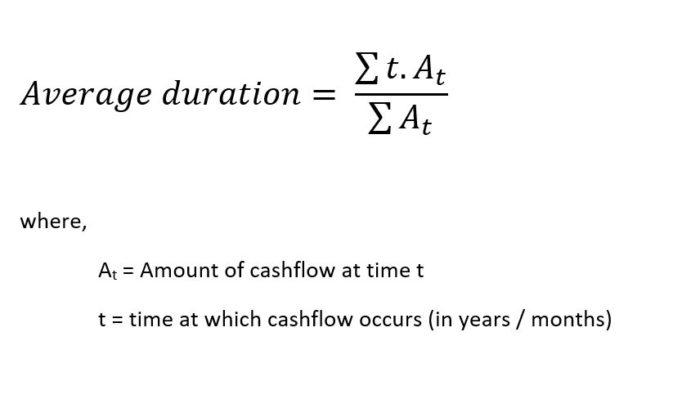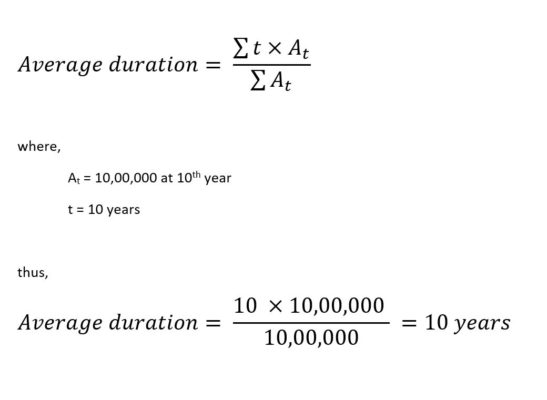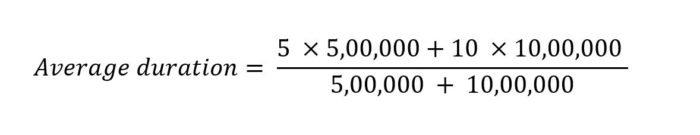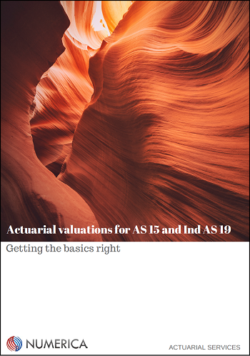We are often asked questions about what is the “duration of liability” and how it impacts the discount rate use in the actuarial valuation of gratuity and leave encashment. We try to cover a few of these questions in this article.

### What is duration of liability?

Duration of liability can be defined in more than one way. However, in actuarial valuation, duration of liability generally refers to the average duration (or mean term) of the liability. In other words, it refers to the average number of years at which a liability is paid off. Please note, the key term is the “average”, i.e. there will be cashflows both before and after this period, but we consider the average.

### Why do we need to calculate duration?

In the context of actuarial valuation, we need to calculate duration to select the discount rate. The discount rate is based on the prevailing interest rates in the market, which vary by duration.

Various accounting standards such as AS 15, Ind AS 19, IAS 19 and US GAAP require that the discount rate should be selected with reference to the duration of liability. You can find more details about selection of the discount rate here.

### How is the duration calculated?

It is the weighted average of the duration of the cashflows, where the future cashflows are used as weights. The formula we use is:#### Example 1:

Let us assume there is a Rs 10 lakhs gratuity due to be paid in 10 years time. Using our formula:Thus, Duration of liability = 10 years

#### Example 2:

Let us assume :

• There is a Rs 10 lakhs gratuity to be paid to an employee retiring at the end of 10 years,
• And another Rs 5 lakhs gratuity to be paid to an employee retiring at the end of 5 years.

As an expectation, the duration of liability should be between 5 and 10 years. Also, since most of the cashflow occurs at 10th year, the duration of liability should be close to 5 years. Let’s see the actual value using our formula.Solving the above equation gives average duration = 8.3 years. As expected, the above value lies between 5 and 10 years, but closer to 10 years, since majority of the cashflow occurs at 10th year.

### How does this impact discount rate?

Generally, an actuary chooses the discount rate based on the duration of liability. From a given yield curve, we choose discount rate corresponding to the time (in years) indicated by duration of liability.

#### Example:

We source our yield curve from CCIL. Let’s say we want to look at yield curve as at 31st December 2018, so we make appropriate date selections, download the excel sheet for 31st December 2018 and then in ZCYC comparison tab, look at the row which says 8.5 years (closest to 8.3 years). The discount rate corresponding to this will thus be 7.46% .

To know more about discount rate, read our article on FAQs about discount rate for actuarial valuation.

### So, does that mean discount rate can be different between gratuity and leave encashment?

That is absolutely correct. Though the underlying member profile may be the same between gratuity and leave encashment, the cashflows timing and amount may vary (in some cases, significantly).

As such, the average duration of liability might differ. Based on the yield curve, this difference in duration of liability might imply a different discount rate for actuarial valuation of gratuity vs. leave encashment.

The difference is not limited to gratuity and leave encashment schemes alone. We should select the discount rate for each employee benefit scheme based on the duration of liability for that scheme.

Many accountants and auditors believe that the discount rate should remain the same for different employee benefit schemes of the same company. This approach is not correct, unless the actuary first aggregates the cashflows from all benefit schemes and then use them to calculate an aggregate duration before selecting the discount rate. However, such an approach will be overly complicated. Just using different discount rates is a much easier option.

Some actuaries set the discount rate with reference to “expected future service” rather than duration of liability. The expected future service remains the same as long as the underlying membership profile of different schemes is the same. This gives them the same discount rate for all schemes. We believe that the discount rate should be selected only on the basis of duration of liability and not the expected future service. Using duration of liability is more closely aligned with the intent behind the accounting standards.

To know more about the basics of actuarial valuation, download our basics guide by clicking on the picture below: